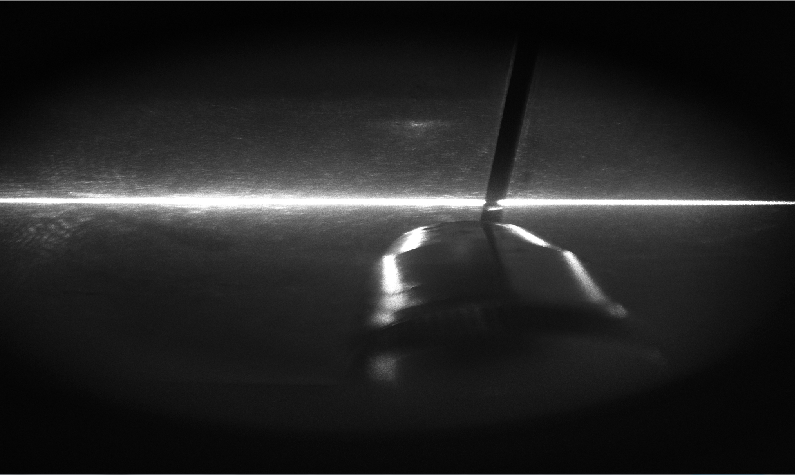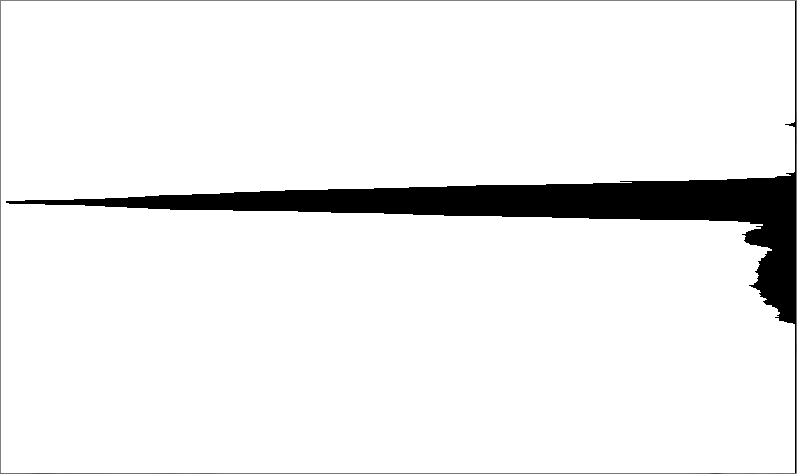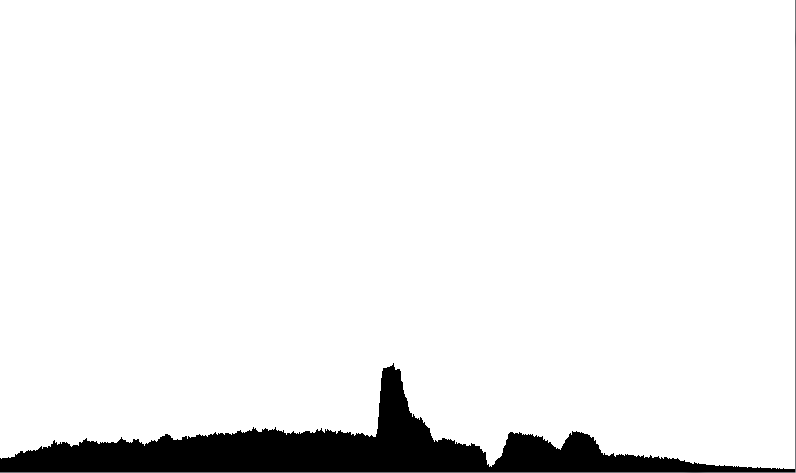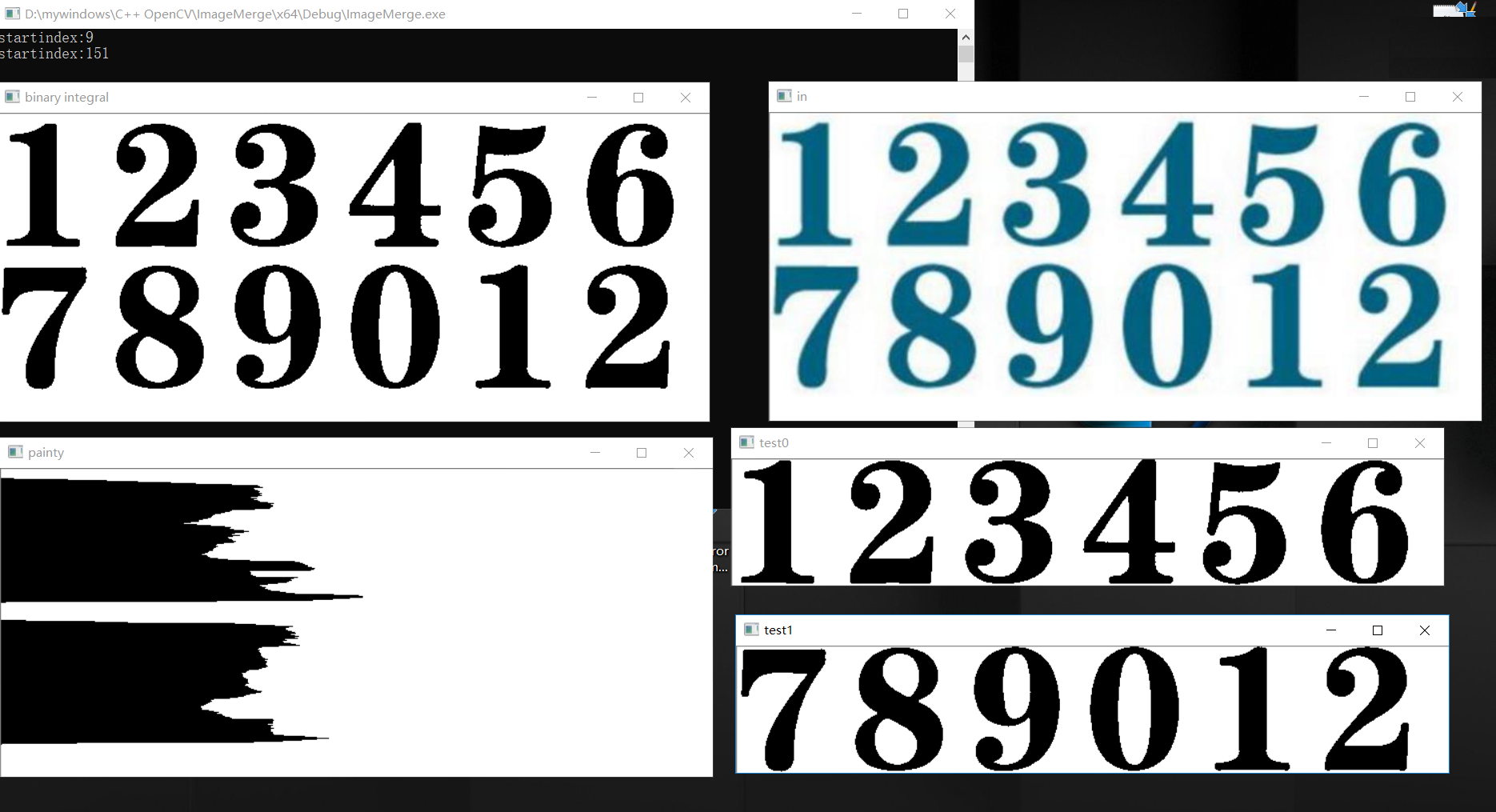水平投影图像处理_数字图像处理水平投影法是什么 - CSDN
• 本文介绍使用opencv实现计算一幅图像水平投影和垂直投影，代码如下：class HorizontalProjection { private: int maxLine;//255最大行位置 int maxNum;//255最多的数目 int minLine;//0最大行位置 int minNum;...
本文介绍使用opencv实现计算一幅图像的水平投影和垂直投影，代码如下：

class HorizontalProjection
{
private:
int maxLine;//255最大行位置
int maxNum;//255最多的数目
int minLine;//0最大行位置
int minNum;//0最多的数目
public:
HorizontalProjection();//构造函数
cv::Mat getHorProjImage(const cv::Mat &image, int threshold);//计算图像的水平投影，并返回一幅图像
};

//构造函数
HorizontalProjection::HorizontalProjection()
{
maxLine = 0;//255最大行位置
maxNum = 0;//255最多的数目
minLine = 0;//0最大行位置
minNum = 0;//0最多的数目
}

//计算图像的水平投影，并返回一幅图像
cv::Mat HorizontalProjection::getHorProjImage(const cv::Mat &image, int threshold)
{
cv::Mat matTmp = image.clone();

if (image.channels() > 1)
{
cvtColor(matTmp, matTmp, CV_RGB2GRAY);//彩色图转为灰度图
}

maxLine = 0, maxNum = 0;//重置255最大数目和最大行
minLine = 0, minNum = matTmp.cols;//重置255最小数目和最小行
int height = matTmp.rows, width = matTmp.cols;//图像的高和宽
int tmp = 0;//保存当前行的255数目
int *projArray = new int[height];//保存每一行255数目的数组
cv::threshold(matTmp, matTmp, threshold, 255, CV_THRESH_BINARY);//对图形进行二值化处理

//循环访问图像数据，查找每一行的255点的数目
for (int i = 0; i < height; ++i)
{
tmp = 0;
for (int j = 0; j < width; ++j)
{
if (matTmp.at<uchar>(i, j) == 255)
{
++tmp;
}
}
projArray[i] = tmp;
if (tmp > maxNum)
{
maxNum = tmp;
maxLine = i;
}
if (tmp < minNum)
{
minNum = tmp;
minLine = i;
}
}

//创建并绘制水平投影图像
cv::Mat projImg(height, width, CV_8U, cv::Scalar(255));

for (int i = 0; i < height; ++i)
{
cv::line(projImg, cv::Point(width - projArray[i], i), cv::Point(width - 1, i), cv::Scalar::all(0));
}

delete[] projArray;//删除new数组
return  projImg;
}

结果如下：水平投影图：垂直投影的代码如下：

class VerticalProjection
{
private:
int maxCol;//255最大行位置
int maxNum;//255最多的数目
int minCol;//0最大行位置
int minNum;//0最多的数目
public:
VerticalProjection();//构造函数
cv::Mat getVerProjImage(const cv::Mat &image, int threshold);//计算图像的水平投影，并返回一幅图像
};

//构造函数
VerticalProjection::VerticalProjection()
{
maxCol= 0;//255最大行位置
maxNum = 0;//255最多的数目
minCol = 0;//0最大行位置
minNum = 0;//0最多的数目
}

//计算图像的水平投影，并返回一幅图像
cv::Mat VerticalProjection::getVerProjImage(const cv::Mat &image, int threshold)
{
cv::Mat matTmp = image.clone();

if (image.channels() > 1)
{
cvtColor(matTmp, matTmp, CV_RGB2GRAY);//彩色图转为灰度图
}
if (threshold == -1)
{
threshold = GetThresholdByOtsu(matTmp);//使用大津算法计算阈值
}

maxCol = 0, maxNum = 0;//重置255最大数目和最大行
minCol = 0, minNum = matTmp.rows;//重置255最小数目和最小行
int height = matTmp.rows, width = matTmp.cols;//图像的高和宽
int tmp = 0;//保存当前行的255数目
int *projArray = new int[width];//保存每一行255数目的数组
cv::threshold(matTmp, matTmp, threshold, 255, CV_THRESH_BINARY);//对图形进行二值化处理

cv::namedWindow("matTmp", 0);
cv::imshow("matTmp", matTmp);

//循环访问图像数据，查找每一行的255点的数目
for (int col = 0; col < width; ++col)
{
tmp = 0;
for (int row = 0; row < height; ++row)
{
if (matTmp.at<uchar>(row, col) == 255)
{
++tmp;
}
}
projArray[col] = tmp;
if (tmp > maxNum)
{
maxNum = tmp;
maxCol = col;
}
if (tmp < minNum)
{
minNum = tmp;
minCol = col;
}
}
//创建并绘制垂直投影图像
cv::Mat projImg(height, width,  CV_8U, cv::Scalar(255));

for (int col = 0; col < width; ++col)
{
cv::line(projImg, cv::Point(col, height - projArray[col]), cv::Point(col, height - 1), cv::Scalar::all(0));
}

delete[] projArray;//删除new数组
return  projImg;
}

结果如下:展开全文opencv
• 经常听到同事说用投影法做图像处理的相关知识，一直没有理解，所以查找资料，这里做一个总结： 投影，在立体几何中我们学到过，是空间直线在某个方向上的投影，那么图像处理中也是这种投影思想。 最简单的投影： ...
经常听到同事说用投影法做图像处理的相关知识，一直没有理解，所以查找资料，这里做一个总结：
投影，在立体几何中我们学到过，是空间直线在某个方向上的投影，那么图像处理中也是这种投影思想。
最简单的投影：
水平方向投影，将图像数组进行列求和；
垂直方向投影，将图像数组进行行求和；
对于二值图像或明显特征的灰度图分割前景与背景，经常用到投影法。

展开全文• 这是一个对二值图像进行垂直和水平方向积分投影的Opencv程序，在VC6.0上运行
• 最近看图像处理反向投影，看到几本书上说的都不是很清楚，自己也不是十分的理解。就在网上找资料，终于找到了一个说的非常直白清楚的文章：原文。现贴出非常核心的原理： 假设我们有一张100x100的输入图像，有一张...
最近看图像处理反向投影，看到几本书上说的都不是很清楚，自己也不是十分的理解。就在网上找资料，终于找到了一个说的非常直白清楚的文章：原文。

现贴出非常核心的原理：
假设我们有一张100x100的输入图像，有一张10x10的模板图像，查找的过程是这样的：
（1）从输入图像的左上角(0,0)开始，切割一块(0,0)至(10,10)的临时图像；
（2）生成临时图像的直方图；
（3）用临时图像的直方图和模板图像的直方图对比，对比结果记为c；
（4）直方图对比结果c，就是结果图像(0,0)处的像素值；
（5）切割输入图像从(0,1)至(10,11)的临时图像，对比直方图，并记录到结果图像；
（6）重复（1）～（5）步直到输入图像的右下角。

其中第3步用到的进行直方图对比方法应该就是标准的哪几种标准的直方图对比方法。（也可以自己定义，即本着越相似值越大的原则就可以）。在Opencv中可以用compareHist（）函数进行直方图对比，当然，我想在opencv中反向投影是默认选择了一种直方图对比方法，因为其函数calcBackProject（）并没有提供选择直方图对比的算法。知道了此原理后，自己实现反向投影也会比较简单。

其实相当于直方图匹配。

参考资料：

http://blog.csdn.net/yee_yj/article/details/6035913
Opencv3编程入门

展开全文• 对于印刷体图片来说，进行水平投影和垂直投影可以很快的进行分割，本文就在OpenCV中如何进行水平投影和垂直投影通过代码进行说明。 水平投影：二维图像在y轴上的投影 垂直投影：二维图像在x轴上的投影 由于投影的...
对于印刷体图片来说，进行水平投影和垂直投影可以很快的进行分割，本文就在OpenCV中如何进行水平投影和垂直投影通过代码进行说明。

水平投影：二维图像在y轴上的投影

垂直投影：二维图像在x轴上的投影

由于投影的图像需要进行二值化，本文采用积分二值化的方式，对图片进行处理。

具体代码如下：

//积分二值化
void thresholdIntegral (Mat inputMat, Mat& outputMat)
{

int nRows = inputMat.rows;
int nCols = inputMat.cols;

// create the integral image
Mat sumMat;
integral (inputMat, sumMat);

int S = MAX (nRows, nCols) / 8;
double T = 0.15;

// perform thresholding
int s2 = S / 2;
int x1, y1, x2, y2, count, sum;

int* p_y1, *p_y2;
uchar* p_inputMat, *p_outputMat;

for (int i = 0; i < nRows; ++i)
{
y1 = i - s2;
y2 = i + s2;

if (y1 < 0)
{
y1 = 0;
}
if (y2 >= nRows)
{
y2 = nRows - 1;
}

p_y1 = sumMat.ptr<int> (y1);
p_y2 = sumMat.ptr<int> (y2);
p_inputMat = inputMat.ptr<uchar> (i);
p_outputMat = outputMat.ptr<uchar> (i);

for (int j = 0; j < nCols; ++j)
{
// set the SxS region
x1 = j - s2;
x2 = j + s2;

if (x1 < 0)
{
x1 = 0;
}
if (x2 >= nCols)
{
x2 = nCols - 1;
}

count = (x2 - x1)* (y2 - y1);

// I(x,y)=s(x2,y2)-s(x1,y2)-s(x2,y1)+s(x1,x1)
sum = p_y2[x2] - p_y1[x2] - p_y2[x1] + p_y1[x1];

if ((int) (p_inputMat[j] * count) < (int) (sum* (1.0 - T)))
{
p_outputMat[j] = 0;
}
else
{
p_outputMat[j] = 255;
}
}
}
}
//垂直方向投影
{
Mat paintx (binary.size(), CV_8UC1, Scalar (255)); //创建一个全白图片，用作显示

int* blackcout = new int[binary.cols];
memset (blackcout, 0, binary.cols * 4);

for (int i = 0; i < binary.rows; i++)
{
for (int j = 0; j < binary.cols; j++)
{
if (binary.at<uchar> (i, j) == 0)
{
blackcout[j]++; //垂直投影按列在x轴进行投影
}
}
}
for (int i = 0; i < binary.cols; i++)
{
for (int j = 0; j < blackcout[i]; j++)
{
paintx.at<uchar> (binary.rows-1-j, i) = 0; //翻转到下面，便于观看
}
}
delete blackcout;
imshow ("paintx", paintx);

}
//水平方向投影并行分割
{
//是否为白色或者黑色根据二值图像的处理得来
Mat painty (binary.size(), CV_8UC1, Scalar (255)); //初始化为全白

//水平投影
int* pointcount = new int[binary.rows]; //在二值图片中记录行中特征点的个数
memset (pointcount, 0, binary.rows * 4);//注意这里需要进行初始化

for (int i = 0; i < binary.rows; i++)
{
for (int j = 0; j < binary.cols; j++)
{
if (binary.at<uchar> (i, j) == 0)
{
pointcount[i]++; //记录每行中黑色点的个数 //水平投影按行在y轴上的投影
}
}
}

for (int i = 0; i < binary.rows; i++)
{
for (int j = 0; j < pointcount[i]; j++) //根据每行中黑色点的个数，进行循环
{

painty.at<uchar> (i, j) = 0;
}

}

imshow ("painty", painty);

vector<Mat> result;
int startindex = 0;
int endindex = 0;
bool inblock = false; //是否遍历到字符位置

for (int i = 0; i < painty.rows; i++)
{

if (!inblock&&pointcount[i] != 0) //进入有字符区域
{
inblock = true;
startindex = i;
cout << "startindex:" << startindex << endl;
}
if (inblock&&pointcount[i] == 0) //进入空白区
{
endindex = i;
inblock = false;
Mat roi = binary.rowRange (startindex, endindex+1); //从而记录从开始到结束行的位置，即可进行行切分
result.push_back (roi);
}
}

for (int i = 0; i < result.size(); i++)
{
Mat tmp = result[i];
imshow ("test"+to_string (i), tmp);
}
delete pointcount;

}
int main (int argc, char* argv[])
{

if (src.empty())
{
return -1;
}

imshow("in",src);

Mat gray;

if (src.channels() == 3)
{
cv::cvtColor (src, gray, CV_BGR2GRAY);
}
else
{
gray = src;
}

Mat bw2 = Mat::zeros (gray.size(), CV_8UC1);
thresholdIntegral (gray, bw2);

cv::imshow ("binary integral", bw2);

waitKey (0);

return 0;
}

输入图片：二值图片：水平投影：垂直投影：行切割：该处理方法，对印刷体有较好的效果，因为印刷体的行列区分明显，因此可以很快的进行行与列的分割。

///////////////////// 2019-01-10From: https://www.linuxidc.com/Linux/2018-01/150264.htm


展开全文• 因为要做图像处理方面的工作，所以最近在学习OpenCv的使用，学习了OpenCv中Mat对象的相关使用之后，实现了使用Mat对象来进行图像的水平投影和垂直投影，并且在投影之后，对字符进行相对应的切分。现在将相关代码贴出...opencv 水平投影
• opencv学习---计算图像水平积分投影和垂直积分投影 标签： opencv水平积分投影垂直积分投影 2016-12-07 18:48 1806人阅读 评论(1) 收藏 举报 分类： opencv学习笔记（1） 作者...
•C#图像镜像
• 什么是数字图像处理？历史、以及它所研究的内容。 说起图像处理，你会想到什么？你是否真的了解这个领域所研究的内容。纵向来说，数字图像处理研究的历史相当悠久；横向来说，数字图像处理研究的话题相当广泛。 ...图像处理 学习方法
• 首先介绍算法思路： matlab代码实现： python代码实现： java代码实现：
• 数字图像处理第五章数字图像处理---几何变换与图像标准（一）点变换（二）仿射变换（三）投影变换（四）应用于图像的几何变换（五）MATLAB 中的图像坐标系统5.1 输出图像位置5.2 控制输出网格（六）图像内插6.1 二维...仿射变换 投影变换
• 基于FPGA水平垂直投影法（字符分割）的实现 作者：OpenSLee 1 背景知识 图像对应方向的投影，就是在该方向取一条直线，统计垂直于该直线（轴）的图像上的像素的黑点数量，累加求和作为该轴该位置的值；基于图像...字符分割
• 一、话说反向投影图像反向投影的最终目的是获取ROI然后实现对ROI区域的标注、识别、测量等图像处理与分析，是计算机视觉与人工智能的常见方法之一。图像反向投影通常是彩色图像投影效果会比灰度图像效果要好，原因...
• Java OpenCV-4.0.0 图像处理21 直方图反向投影 Java OpenCV-4.0.0 直方图反向投影 反向投影是反映直方图模型在目标图像中的分布情况。 简单点说就是用直方图模型去目标图像中寻找是否有相似的对象。通常用HSV色彩...直方图反向投影 OpenCV Java
• 数字图像处理最早出现于20世纪50年代，当时的电子计算机已经发展到一定水平，人们开始利用计算机来处理图形和图像信息。数字图像处理作为一门学科大约形成于20世纪60年代初期。早期的图像处理的目的是改善图像的质量...
• 在上篇博客中，我们已经实现了水平投影和垂直投影图的绘制。接下来，我们可以根据获得的投影数据进行图像的分割，该法用于文本分割较多，所以此处依然以上次的图为例。 先把上次的两幅图搬过来，方便讲解。 上面两...Python 分割 阈值
• 我们所说的图像处理实际上就是数字图像处理，是把真实世界中的连续三维随机信号投影到传感器的二维平面上，采样并量化后得到二维矩阵。数字图像处理就是二维矩阵的处理，而从二维图像中恢复出三维场景就是计算机视觉...
• 代码实现简单验证码检测，由于识别库比较难配，尴尬，没有成功~不过没有关系，图像处理的算法实现了就可以了。 原图： 使用投影分割，先竖直方向，后水平方向，基本方法就是遍历像素点，源代码： int otsu...
...